# Lesson 3 learning objectives

At the end of this lesson, students will …

• Understand the difference between a linear model and a linear mixed model.
• Understand the difference between random intercepts and random slopes.
• Be able to fit a mixed model with fixed effects, both continuous and categorical, and interactions.
• Be able to fit a mixed model with random effects, both random intercepts and random slopes.
• Examine diagnostic plots to ensure that model assumptions are met.
• Examine summary information from mixed model output, including ANOVA tables.

For this lesson, we will keep it simple and use simulated data. First load the packages we will need for this lesson. Then read the `soilN_biomass.csv` file, creating a data frame called `soilN_biomass`. This dataset might have come from a study where there are five different fields, each of which has 20 plots. In each plot, soil nitrogen and plant biomass is measured.

``````library(tidyverse)
library(lme4)
library(lmerTest)
library(easystats)``````
``soilN_biomass <- read_csv('https://usda-ree-ars.github.io/SEAStats/mixed_models_in_R/datasets/soilN_biomass.csv')``
``````## Rows: 100 Columns: 3
## ── Column specification ────────────────────────────────────────────────────────
## Delimiter: ","
## chr (1): field
## dbl (2): soilN, biomass
##
## ℹ Use `spec()` to retrieve the full column specification for this data.
## ℹ Specify the column types or set `show_col_types = FALSE` to quiet this message.``````

Let’s look at the data. Use functions you learned in lesson 2.

``````soilN_biomass

summary(soilN_biomass)

glimpse(soilN_biomass)``````

There is a character column to identify which field the measurement comes from, a numeric column for the soil N value, and a numeric column for the plant biomass value.

# Plot the data

Here is a plot made using the ggplot2 package. Don’t worry about the code used to make this plot for now.

``````ggplot(soilN_biomass, aes(x = soilN, y = biomass, color = field)) +
geom_point(size = 1.5) +
theme_bw()``````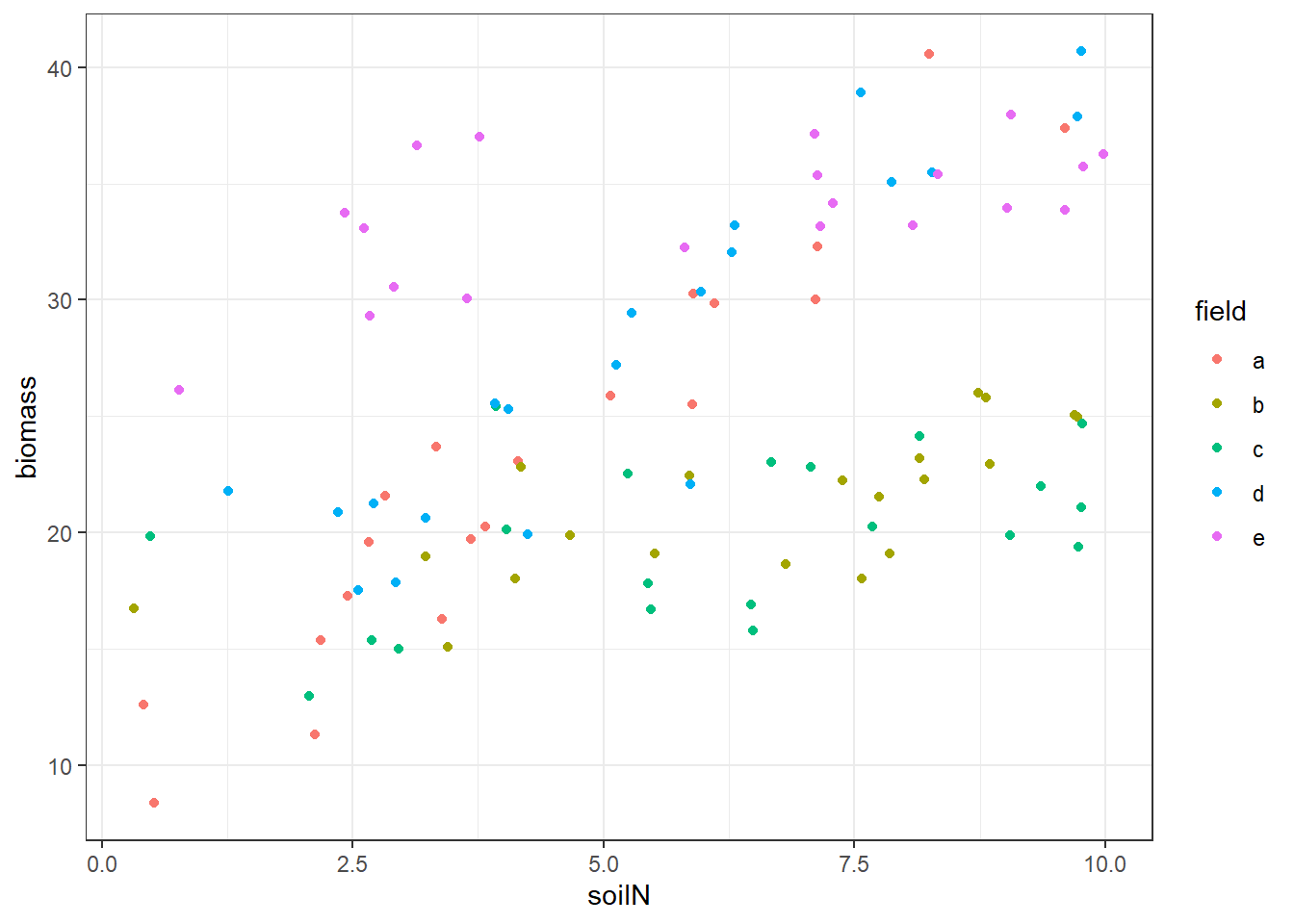You can see from the plot that the relationship between soil N and plant biomass is sort of unclear. Let’s look at how we might fit a model to this dataset.

# Model Syntax in R

The bare minimum we need to specify in R to fit a model is the model formula and the data frame that the variables in the model formula come from.

The formula consists of a left-hand side (LHS), a tilde `~`, and a right-hand side (RHS).

The left-hand side is the name of the response variable (y). In this lesson we are only going to be focusing on models with a single response variable.

The right-hand side contains the predictor variables (x). Effects are separated by a plus sign `+`. Interaction effects are denoted with a colon `:`. The shorthand `*` indicates all possible combinations of interactions for a set of variables. I do not recommend using this shorthand but you may see it. Another shorthand is `.` which means all variables in the dataset other than the y variable.

We will cover random effect syntax later.

So a typical formula might be

`weight ~ height + sex + height:sex`

(In SAS this would be `model weight = height sex height*sex`). This means the expected value of weight is a linear combination of height (a continuous variable), sex (a binary variable), and the product or interaction of height and sex.

By default, a model includes an intercept term without you having to explicitly specify one. You can include a `1` in the right-hand side if you want to be explicit that the model includes an intercept:

`weight ~ 1 + height`

By contrast, if you want to fit a model without an intercept, “forcing” the regression line to pass through the origin, you can do it by replacing the `1` with a `0` like this:

`weight ~ 0 + height`

Finally, the `data` argument tells the model fitting function what data frame contains the variables in the model formula.

# Linear model with continuous predictor

Let’s say we want to know the relationship between soil nitrogen in a plot and the biomass of plants in the plot. We might start by fitting a simple linear regression model, ignoring the field that each plot is located in.

We use the `lm()` (linear model) function for this. The model formula is very simple.

``lm_fit <- lm(biomass ~ soilN, data = soilN_biomass)``

You can use the `summary()` function to look at the model output.

``summary(lm_fit)``
``````##
## Call:
## lm(formula = biomass ~ soilN, data = soilN_biomass)
##
## Residuals:
##     Min      1Q  Median      3Q     Max
## -11.147  -5.487  -1.199   5.302  14.912
##
## Coefficients:
##             Estimate Std. Error t value Pr(>|t|)
## (Intercept)  17.5108     1.5376  11.389  < 2e-16 ***
## soilN         1.3364     0.2452   5.449 3.77e-07 ***
## ---
## Signif. codes:  0 '***' 0.001 '**' 0.01 '*' 0.05 '.' 0.1 ' ' 1
##
## Residual standard error: 6.635 on 98 degrees of freedom
## Multiple R-squared:  0.2325, Adjusted R-squared:  0.2247
## F-statistic:  29.7 on 1 and 98 DF,  p-value: 3.768e-07``````

We can see that there is a positive coefficient of ~1.34 on `soilN` indicating that the simple regression finds that for every 1 unit increase in soil N, there is a 1.34 unit increase in biomass.

If you just want the coefficients you can use the `coef()` function:

``````lm_coefs <- coef(lm_fit)

lm_coefs``````
``````## (Intercept)       soilN
##   17.510752    1.336415``````

But what does the fitted trend look like? We will recreate the plot we made previously but now include a fitted line with the intercept and slope coefficients from the model.

``````ggplot(soilN_biomass, aes(x = soilN, y = biomass, color = field)) +
geom_point(size = 1.5) +
theme_bw() +
geom_abline(intercept = lm_coefs, slope = lm_coefs, size = 1)``````
``````## Warning: Using `size` aesthetic for lines was deprecated in ggplot2 3.4.0.
## This warning is displayed once every 8 hours.
## Call `lifecycle::last_lifecycle_warnings()` to see where this warning was
## generated.``````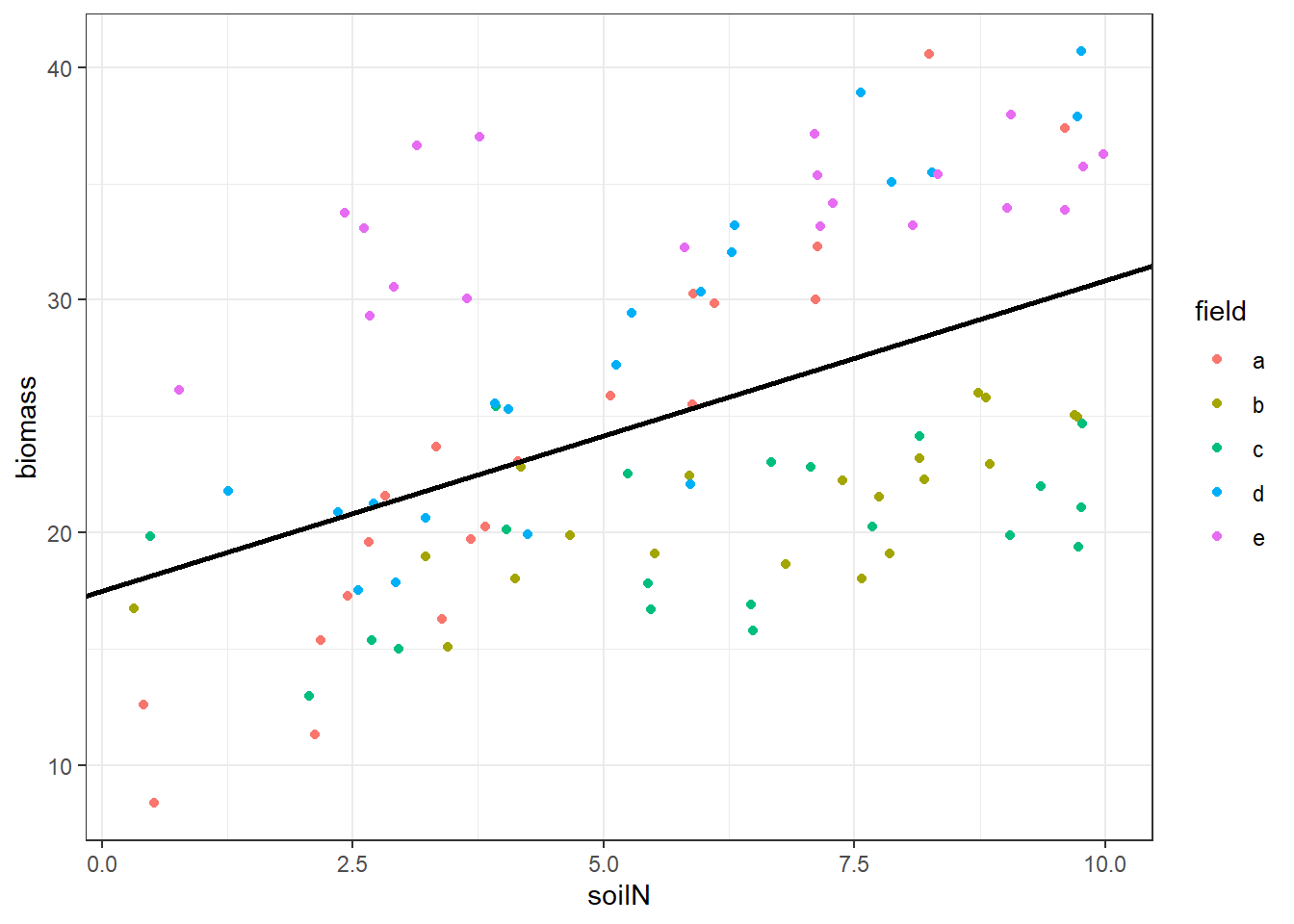The fit is relatively poor because the linear regression assumes that a single relationship, with the same intercept and slope, holds across all fields. However, this might not be a good assumption. Fields might have different unmeasured environmental conditions that cause them to have different relationships between soil nitrogen and biomass. For instance, some fields might have higher soil phosphorus, or better soil moisture conditions – or the variation might even be completely random.

Also, there are five fields, each with 20 measurements. The measurements that come from the same field are not independent because they have a common cause: whatever properties the soil in field A has that influence biomass are affecting all the biomass measurements from field A in the same way. Our call of `summary(lm_fit)` above showed that the model had 98 degrees of freedom (100 data points - 2 parameters, the intercept and slope = 98). 98 degrees of freedom is probably too many. But if we just average all the measurements from each field into a single measurement, we will only have 3 degrees of freedom in the model. A more appropriate value would be somewhere in between – the measurements from the same barnyard are partially, but not completely, dependent on one another.

# Separate linear regressions

OK, so we are missing something if we lump all the fields together, because the relationship between soil N and plant biomass may be different in each field. Well then, you might say, why not fit separate models for each field?

I will demonstrate how you might do this – note this code is a little bit more complex than what we’ll be covering in this workshop so take this more as a demonstration. It is not something you would typically want to do. We’re fitting five separate linear regression models, estimating an intercept and slope for each field, and then pulls out the coefficients and makes a plot of the fitted lines.

``````separate_lm_fits <- soilN_biomass %>%
group_by(field) %>%
group_map(~ lm(biomass ~ soilN, data = .))

separate_lm_coefs <- data.frame(field = c('a', 'b', 'c', 'd', 'e'),
intercept = map_dbl(separate_lm_fits, ~ coef(.)),
slope = map_dbl(separate_lm_fits, ~ coef(.)))

separate_lm_coefs``````
``````##   field intercept     slope
## 1     a  8.834202 3.2808603
## 2     b 15.185729 0.9094639
## 3     c 16.219772 0.5811871
## 4     d 13.204062 2.7437149
## 5     e 30.170463 0.5936931``````
``````ggplot(soilN_biomass, aes(x = soilN, y = biomass, color = field)) +
geom_point(size = 1.5) +
theme_bw() +
geom_abline(aes(intercept = intercept, slope = slope, color = field), size = 1, data = separate_lm_coefs)``````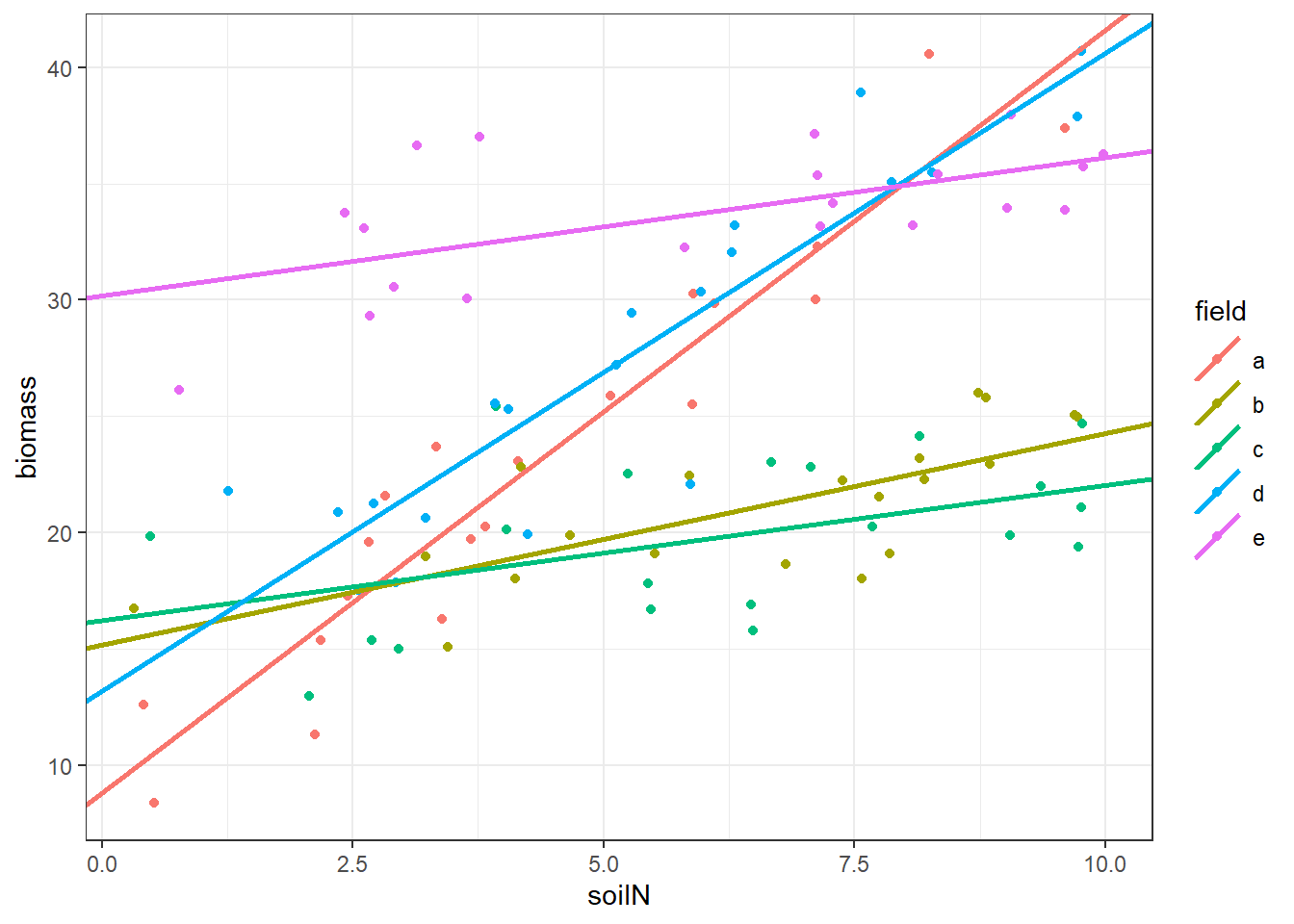That is an improvement because we now have a separate relationship for each field. But we are still missing something: we believe that there is some overall tendency of soil N to affect plant biomass, a process that is occurring in every field but has different outcomes because of the different environments in each field. How do we account for this?

# Our first linear mixed model

It might seem like we have dealt with the issue that the different fields might have different relationships between soil nitrogen and plant biomass. But we went a little bit too far. We really want to know how soil nitrogen affects plant biomass in general, not in one particular field … the goal of our scientific inference is usually to generalize what we find in a particular study to a larger population of fields (only a few of which we actually visited to get soil N and plant biomass measurements in our study). Doing separate regressions for each field doesn’t quite get us there because it doesn’t give us an estimate of the overall relationship between soil nitrogen and plant biomass in fields, while at the same time accounting for variation due to the unknown factors that may be different from field to field.

How can we solve that problem? You probably already guessed it … a mixed model! I’m calling these mixed models but they go by a lot of other names.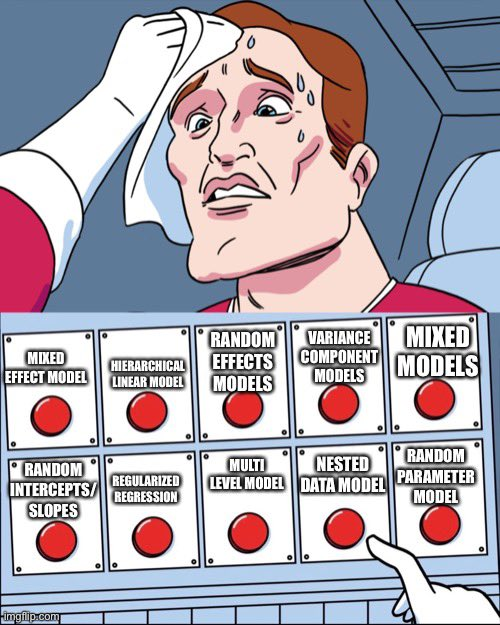Mixed models meme courtesy of Chelsea Parlett-Pelleriti on Twitter

Mixed models do what is called “partial pooling.” This means they don’t lump all observations together into a single group (“Complete pooling” as we did in the simple linear regression above). Instead, they “partially” group them: some of the parameters, the fixed effects, are shared by all the groups, but other parameters, the random effects, are unique to each group. They’re called mixed models because they have a mix of fixed and random effects.

## Random intercept model

Here’s our first mixed model. We are now using the `lmer()` function from the lme4 package instead of `lm()` from base R.

``mm_fit <- lmer(biomass ~ soilN + (1 | field), data = soilN_biomass)``

This looks just like the `lm()` call except for the `+ (1 | field)` part. What is that? It indicates that a separate random intercept will be fit to each field. The random effect term is contained within parentheses `()` and has a vertical bar `|` in the middle of it. To the left of the `|` is the design component, where the `1` indicates an intercept. To the right are the grouping factors; here it is only `field`. We will see more complicated random-effects specifications later.

There are a lot of other mixed model fitting packages in R besides lme4 but it is a very good one for basic models like the ones we will be covering in this workshop.

## Visualizing model fit of random intercept model

What result do we get? First let’s look at a plot of the results. Again, this code is for demonstration purposes only for now. This plot includes a thin line for each of the group-level predictions (separate for each field) as well as a thick black line for the population-level prediction (average expected value across all fields).

``````pred_grid <- expand.grid(soilN = c(0, 10), field = letters[1:5])
mm_pred_group <- cbind(pred_grid, biomass = predict(mm_fit, newdata = pred_grid))
mm_pred_population <- data.frame(soilN = c(0, 10), biomass = predict(mm_fit, newdata = data.frame(soilN = c(0, 10)), re.form = ~ 0))

ggplot(soilN_biomass, aes(x = soilN, y = biomass, color = field)) +
geom_point(size = 1.5) +
theme_bw() +
geom_line(data = mm_pred_group) +
geom_line(data = mm_pred_population, color = 'black', size = 1)``````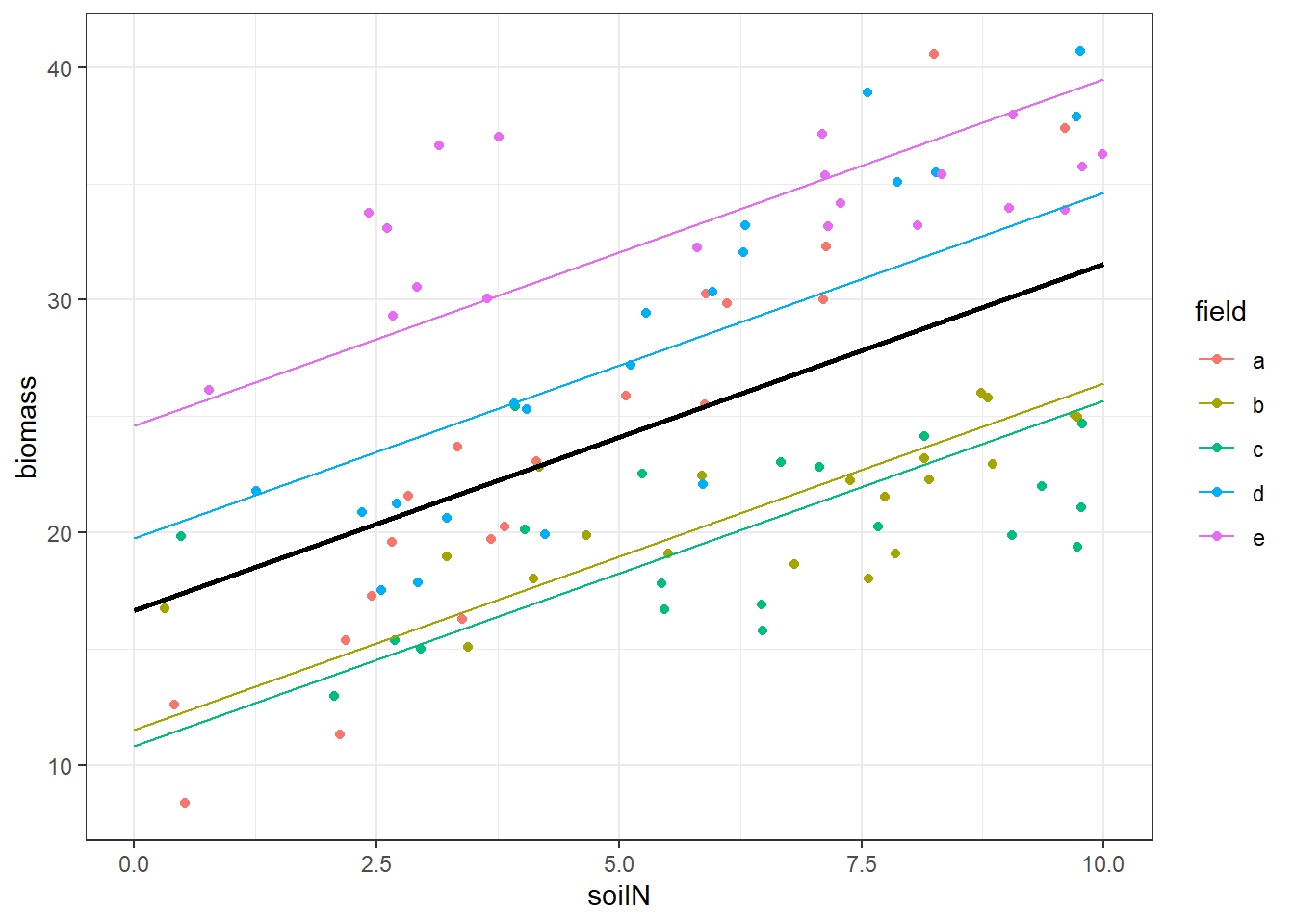This model has accounted for different overall biomass levels in each field: the intercepts of each of the five lines are different. The thick black line shows the population-level expectation for the “average” field. The mixed model fits the intercepts of each field as if they were drawn from a normal distribution, and the population-level prediction’s intercept is the mean of that distribution. But this model still does not allow for there to be a different trend in biomass as soil nitrogen increases within each field: the lines are all parallel with the same slope.

## Random slope model

We can allow not only the intercepts, but also the slopes, to vary by field. The syntax for that model differs from the random intercept model because the `1`, indicating intercept only, in the design part of the random effects term is replaced with `soilN`, meaning the slope with respect to `soilN` will be different for each grouping factor `field`.

``mm_fit_randomslopes <- lmer(biomass ~ soilN + (soilN | field), data = soilN_biomass)``

## Visualizing model fit of random slope model

Now what does the model fit look like?

``````mm_pred_group_randomslopes <- cbind(pred_grid, biomass = predict(mm_fit_randomslopes, newdata = pred_grid))
mm_pred_population_randomslopes <- data.frame(soilN = c(0, 10), biomass = predict(mm_fit_randomslopes, newdata = data.frame(soilN = c(0, 10)), re.form = ~ 0))

ggplot(soilN_biomass, aes(x = soilN, y = biomass, color = field)) +
geom_point(size = 1.5) +
theme_bw() +
geom_line(data = mm_pred_group_randomslopes) +
geom_line(data = mm_pred_population_randomslopes, color = 'black', size = 1)``````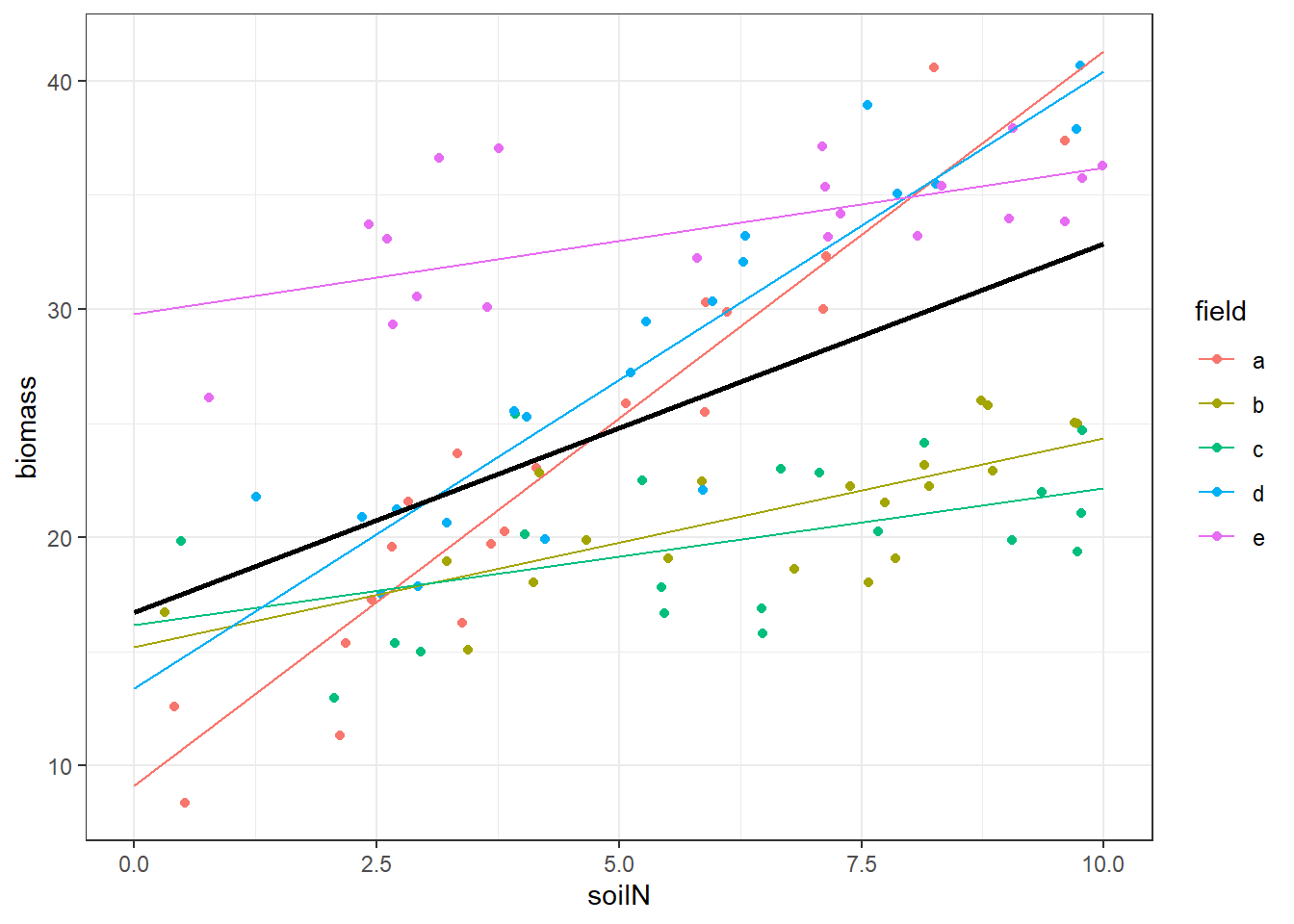Now, we have group-level predictions that capture the different trends within each field, as well as a population-level prediction for the “average” field.

# Delving into mixed model output

Let’s look at some of the ways we can extract output from a fitted mixed model object.

## Diagnostic plots

First let’s take a step back and make sure our statistical model meets all assumptions. For a linear mixed model, this means not only do the residuals need to be roughly normally distributed and have no trend with respect to the fitted values, but also the random effects need to be normally distributed.

I like using the `check_model()` function which is in the performance package that loads automatically when you load the easystats package. It produces a lot of diagnostic plots that you can use to make sure your model meets all the assumptions.

``check_model(mm_fit_randomslopes)``
``````## Not enough model terms in the conditional part of the model to check for
##   multicollinearity.``````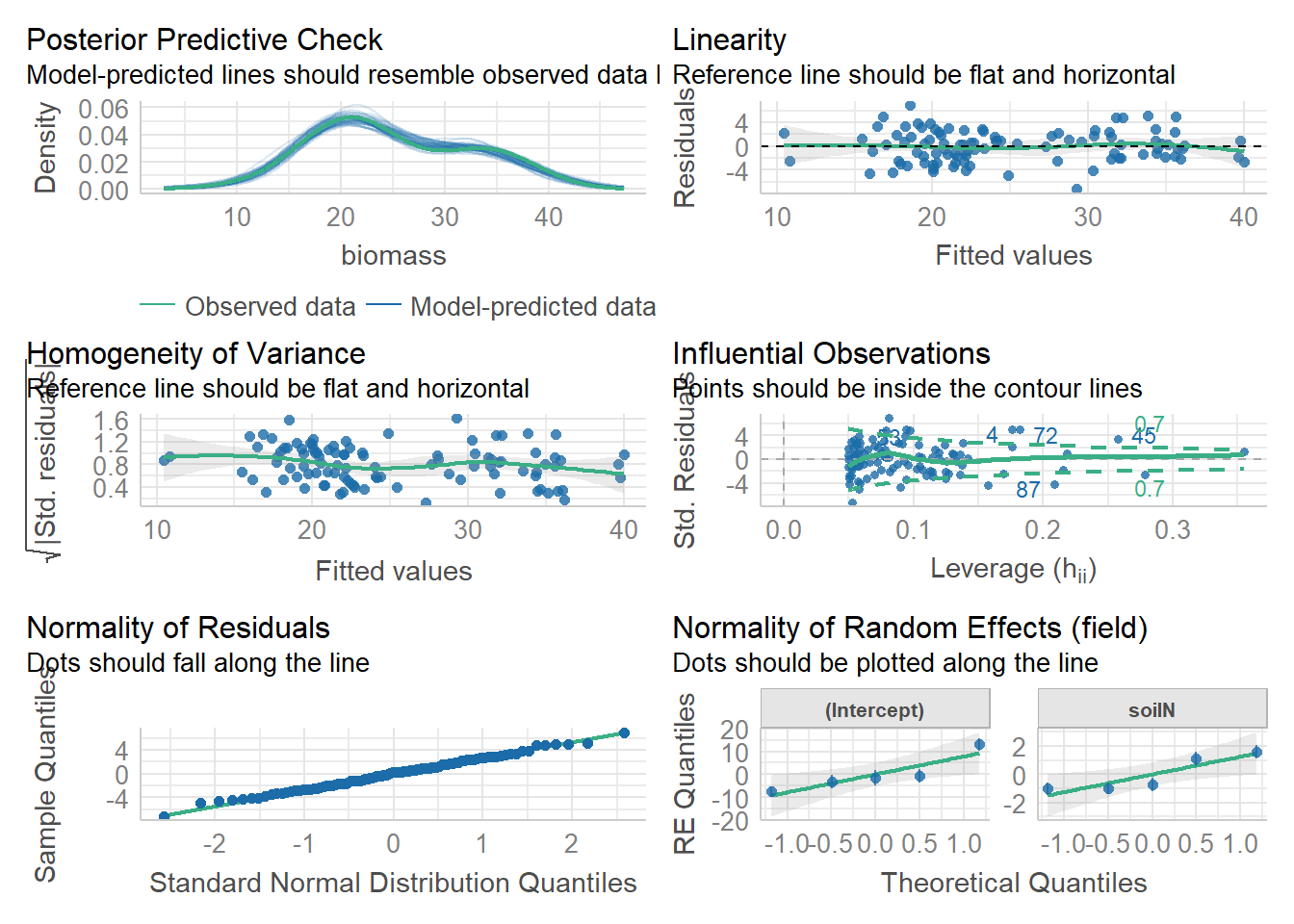These diagnostic plots look great (obviously so, because I simulated this dataset using normal distributions for everything)! The linearity and homogeneity of variance plots show no trend. The normal Q-Q plots for the overall residuals (bottom left) and for the random effects (bottom right) all fall on a straight line so we can be satisfied with that.

I personally do not recommend running any formal tests on residuals such as the Shapiro-Wilk test. Whenever scientists ask me about them, I try to dissuade them. This is because assumptions like the normality of residuals assumption are only approximations. A true perfect normal distribution does not exist in nature. Just like a perfect circle, it is a human construct. There will always be small deviations. For your model to be valid, it just has to be “close enough.” Minor differences between the residuals and the normal distribution are not a problem; we will explore how to use generalized linear mixed models to deal with situations when there are really big discrepancies.

But the most important assumption of any linear model is not one you can test with any of these graphs. People get hung up on these assumptions because you can easily run tests and make plots to check them. But by far the most important assumption for a statistical model of this kind is that individual data points are independent samples. Or if there is any dependence between them, it has to be accounted for. That’s why mixed models are so crucial – they allow us to account for the partial dependency of data points.

## Model summaries

Here are some ways we can look at the quantitative results of the model fit. (Tomorrow we will go over more sophisticated ways of presenting this output.)

We can use `summary()` to get a lot of output. What’s in here? We have information about the distribution of residuals and random effects, and the fixed effect coefficients with t-tests.

``summary(mm_fit_randomslopes)``
``````## Linear mixed model fit by REML. t-tests use Satterthwaite's method [
## lmerModLmerTest]
## Formula: biomass ~ soilN + (soilN | field)
##    Data: soilN_biomass
##
## REML criterion at convergence: 518.6
##
## Scaled residuals:
##      Min       1Q   Median       3Q      Max
## -2.62360 -0.66863  0.08365  0.65974  2.50925
##
## Random effects:
##  Groups   Name        Variance Std.Dev. Corr
##  field    (Intercept) 62.636   7.914
##           soilN        1.609   1.269    -0.70
##  Residual              7.548   2.747
## Number of obs: 100, groups:  field, 5
##
## Fixed effects:
##             Estimate Std. Error      df t value Pr(>|t|)
## (Intercept)  16.7319     3.6014  4.0358   4.646  0.00949 **
## soilN         1.6155     0.5773  3.9919   2.798  0.04902 *
## ---
## Signif. codes:  0 '***' 0.001 '**' 0.01 '*' 0.05 '.' 0.1 ' ' 1
##
## Correlation of Fixed Effects:
##       (Intr)
## soilN -0.707``````

The three functions `ranef()`, `fixef()`, and `coef()` give us the random effects, the fixed effects (population-level intercept and slope), and the coefficients (sum of random and fixed effects, resulting in the group-level intercepts and slopes).

``ranef(mm_fit_randomslopes)``
``````## \$field
##   (Intercept)      soilN
## a  -7.6143594  1.6016461
## b  -1.5412869 -0.6984713
## c  -0.5646425 -1.0160748
## d  -3.3277305  1.0870341
## e  13.0480193 -0.9741341
##
## with conditional variances for "field"``````